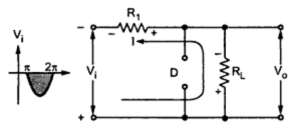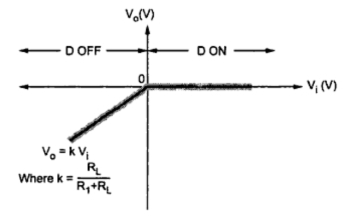### Basic Parallel Clipper with Positive Cliping

Parallel Clippers : Part1
In parallel clipper circuit, the diode is connected across the load terminals. It can be used to clip or limit the positive or negative part of the input signal, as per the requirement.
1.1 Basic Parallel Clipper with Positive Clipping
The Fig. 1 shows the basic parallel clipper circuit in which diode D is connected across the load resistance RL. The resistance R1 is current controlling resistance.Fig. 1  Basic parallel clipper
Operation
Assumed ideal diode. During positive half cycle of the input Vi, the diode D becomes forward biased and remains forward biased for the entire half cycle of the input.
Note : As ideal diode acts as a short circuit when forward biased, the current I flows entirely through diode D. The drop across short circuit diode is zero.
As is in parallel with diode no current flows through it and output voltage Vo = 0V  as shown in the Fig. 2.Fig. 2  Operating during positive half cycle
Note : This condition remains for entire positive half cycle of the input. Thus positive half cycle gets clipped off.
During negative half cycle of input, the diode is reverse biased and acts as open circuit. The entire current flows through RL as shown in the Fig. 3.Fig. 3  Operation during negative half cycle
Hence Vo = (Vi RL )/(RL + R1) using potential divider rule. Thus Vo α  Vi and there exists straight line relationship between the input and output voltage.
Note : As Vo α  Vi during negative half cycle of the input, proportionate negative half cycle is available at the output.
The waveforms are shown in the Fig. 4.Fig. 4  Waveforms for parallel clipper

Transfer characteristics :
The mathematical equations for the transfer characteristics are,
The transfer characteristics are shown in the Fig. 5.Fig. 5 Transfer characteristics of positive parallel clipper

Note : Making R1 <<RL, Vo = Vin can be obtained.